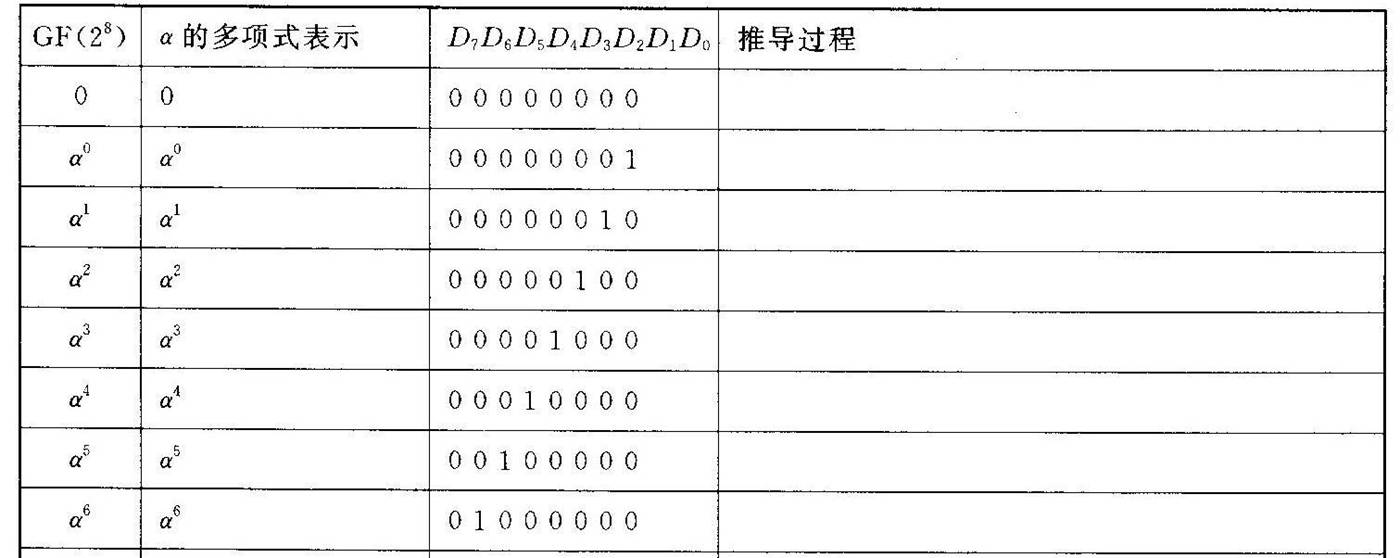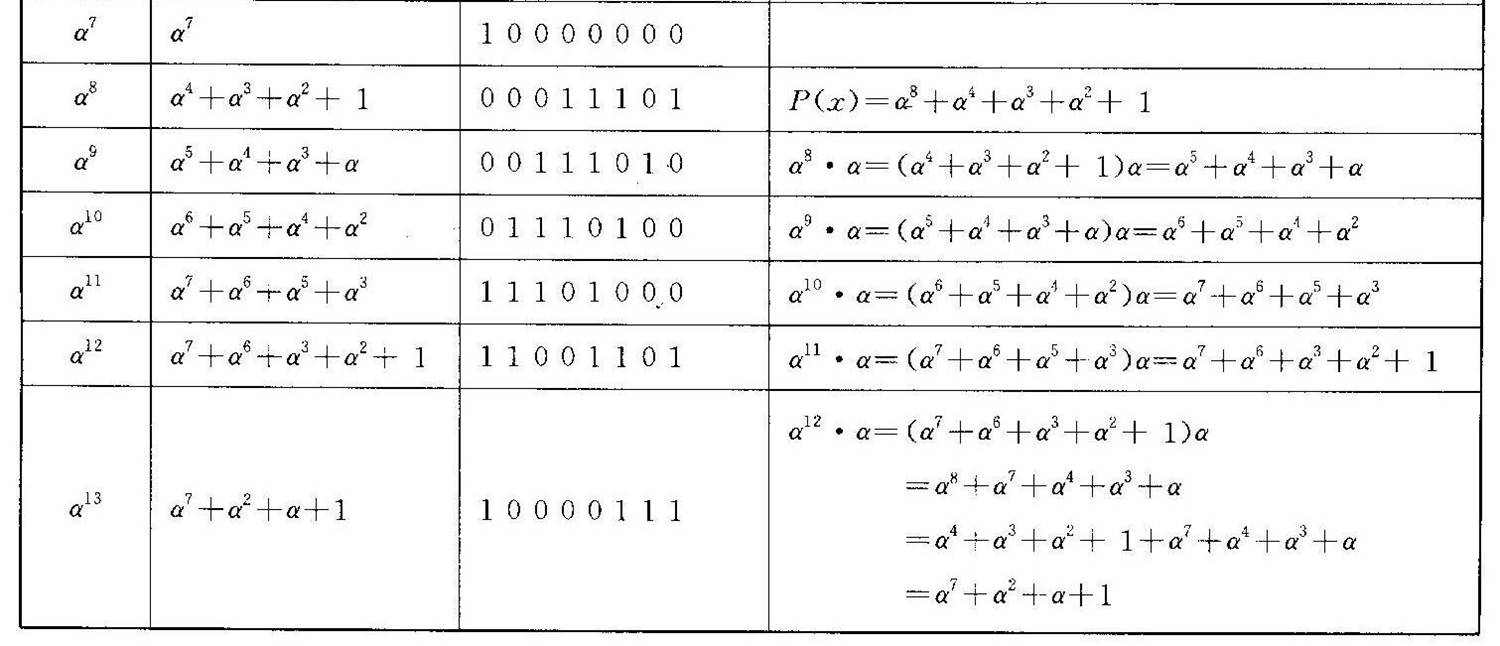# 伽罗华域(Galois Field，GF，有限域)乘法运算

GF(2m)域

α8＋α4＋α3＋α2＋1 = 0
即可以得到 α84＋α3＋α2＋1下面就按以下规则进行乘法运算

0=000   就是0
1=001   就是1
2=0010就是x+0=x
3=0011就是x+1
4=00100就是x^2
然后对于两个变量
u,v
可以先计算两个对应多项式的乘积（需要注意的是加法是模2的，或者说是异或运算），
比如
3*7=(x+1)*(x^2+x+1)=x*x^2+x*x+x+x^2+x+1=x^3+1   (模2运算中x+x=0   and   x^2+x^2=0)
所以3*7=9
在乘积得出来的多项式次数大于7时，我们需要对多项式在GF(2)上关于h(x)求余数，也就是
129*5=(x^7+1)*(x^2+1)=x^9+x^7+x^2+1
将上面的函数加上x*h(x)可以消去x^9,（其实就是手工除法过程，只是现在每一次商总是0或1），所以
129*5=x^9+x^7+x^2+1+x^9+x^5+x^4+x^3+x=x^7+x^5+x^4+x^3+x^2+x+1
=0010111111=191

01-1130
03-197229
12-10501
04-115770
04-29983
07-091157
05-01854
11-28833
01-30569
05-18797
12-21
03-211万+
04-044801
11-276530
08-171万+
05-17437
05-17285

### “相关推荐”对你有帮助么？

•非常没帮助
•没帮助
•一般
•有帮助
•非常有帮助被折叠的  条评论 为什么被折叠?到【灌水乐园】发言MengBoy

¥2 ¥4 ¥6 ¥10 ¥20余额支付 (余额：-- )扫码支付获取中扫码支付点击重新获取扫码支付1.余额是钱包充值的虚拟货币，按照1:1的比例进行支付金额的抵扣。
2.余额无法直接购买下载，可以购买VIP、C币套餐、付费专栏及课程。余额充值